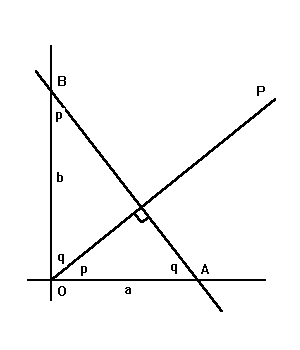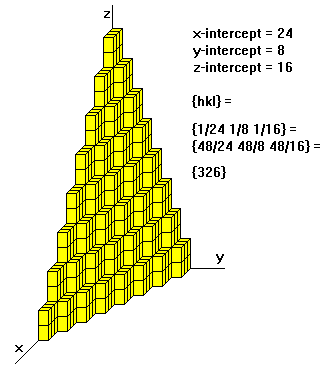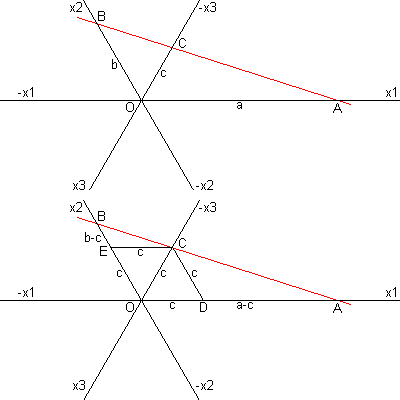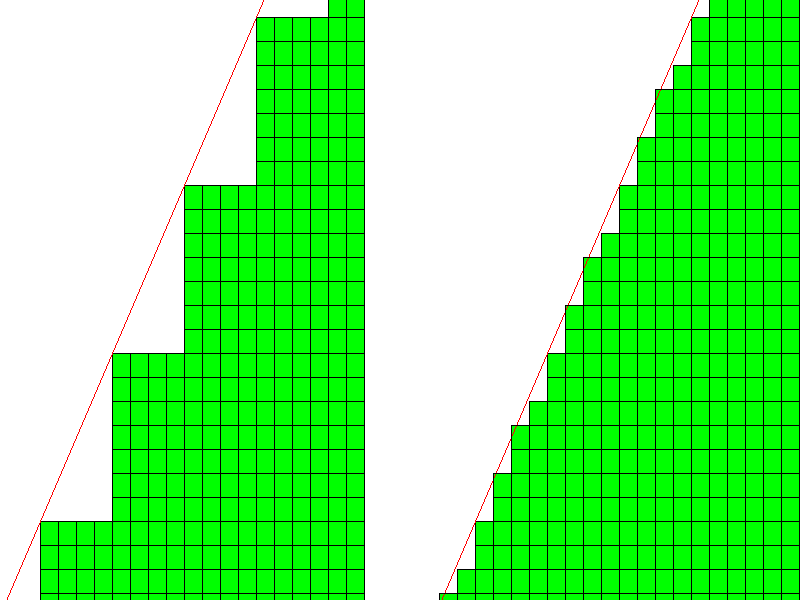# Miller Indices

Steven Dutch, Professor Emeritus, Natural and Applied Sciences, University of Wisconsin - Green Bay

## Lines in the PlaneWhat are the equations of lines AB and OP?. The generic equation of a line is y = mx + b, where b is the y-intercept and m is the slope. For line AB, the slope is -tan q, or -b/a. Thus we have: Line AB: y = -bx/a + b, or, x/a + y/b = 1. If OP is perpendicular to AB, its y- intercept is obviously zero, and we have y = x tan p, or y = xa/b, or xa = yb. Thus, a general line has a simple equation in terms of its intercepts, and a line through the origin perpendicular to the line also has a simple equation in terms of the intercepts. As a general rule, if two lines are perpendicular, the product of their slopes is -1.

## Lines and Planes in Three DimensionsThe equation for a plane in three dimensions is exactly analogous for the line in two dimensions: x/a + y/b + z/c = 1. If line OP is perpendicular to the plane, its equation is also analogous to the two-dimensional case: xa = yb = zc. If we know a plane, we know the line through the origin perpendicular to it, called the pole to the plane. Quantities a, b and c are termed the direction numbers of the line. Line OP makes angles A, B, and C with the axes. The angles are related as follows: cos2 A + cos2 B + cos2 C = 1

Cos A, cos B and cos C are called the direction cosines of the line. They are obviously also direction numbers. If you know any arbitrary direction numbers a, b and c, then cos A = a/s, cos B = b/s and cos c = c/s, where s2 = a2 + b2 + c2

## Miller Indices

Now that we know the equation of a plane in space, the rules for Miller Indices are a little more intelligible. They are:

• Determine the intercepts of the face along the crystallographic axes, in terms of unit cell dimensions.
• Take the reciprocals
• Clear fractions
• Reduce to lowest terms

For example, if the x-, y-, and z- intercepts are 2,1, and 3, the Miller indices are calculated as:

• Take reciprocals: 1/2, 1/1, 1/3
• Clear fractions {multiply by 6}: 3, 6, 2
• Reduce to lowest terms (already there)

Thus, the Miller indices are 3,6,2. If a plane is parallel to an axis, its intercept is at infinity and its Miller index is zero. A generic Miller index is denoted by {hkl}. The convention is to use curly brackets for face indices.If a plane has negative intercept, the negative number is denoted by a bar above the number. Never alter negative numbers. For example, do not divide -1, -1, -1 by -1 to get 1,1,1. This implies symmetry that the crystal may not have!

For hexagonal and trigonal minerals, there are three possible axis directions, spaced 120 degrees apart:Obviously, any two intercepts specify the face. Also, there will be two intercepts of one sign and one of the other. The Miller indices for a hexagonal mineral are often written {hikl}. Indices h, i and k are related by h + i + k = 0. Some modern texts dispense with the i term and treat hexagonal minerals like all others.The proof is simple: Imagine a hexagonal axis system as shown and a face cutting each axis with intercepts a, b, and c. Now construct CE parallel to x1 and CD parallel to x2. Obviously triangles AOB, ADC and CEB are similar. Therefore a/b = {a-c}/c = a/c -1. Dividing by a and rearranging, we have 1/a + 1/b = 1/c. Define h = 1/a, k = 1/b and i = -1/c. Then h + i + k =0.

### Some General Principles

• If a Miller index is zero, the plane is parallel to that axis.
• The smaller a Miller index, the more nearly parallel the plane is to the axis.
• The larger a Miller index, the more nearly perpendicular a plane is to that axis.
• Multiplying or dividing a Miller index by a constant has no effect on the orientation of the plane
• Miller indices are almost always small.

### Why Miller Indices?

• Using reciprocals spares us the complication of infinite intercepts.
• Formulas involving Miller indices are very similar to related formulas from analytical geometry.
• Specifying dimensions in unit cell terms means that the same label can be applied to any face with a similar stacking pattern, regardless of the crystal class of the crystal. Face 111 always steps the same way whether the crystal is isometric or triclinic.## Miller Indices and Lines

The Miller Index of a line is about as simple as it can be: if the line passes through {h, k, l}, its Miller Index is [hkl], written in brackets to distinguish it from a face.

### Zones

A family of faces all parallel to some particular line is called a zone, and the line is called the zone axis. Two faces {hkl} and {pqr} belong to zone [kr-lq, lp-hr, hq-kp]. Note the similarity of this formula to the cross-product formula from vector mechanics. Any other face {def} belongs to the same zone if its indices are some linear combination of {hkl} and {pqr}, for example, d = 2h-3p, e = 2k-3q, etc.

For example, faces {110} and {010} belong to [1*0-0*1, 0*0-1*0, 1*1-1*0], or . The final zero in the face indices is a tipoff that they are both parallel to the z-axis, and the zone index  is the z-axis. Any other face whose index is some linear combination of {110} and {010} is also a member of that zone. Obviously the final index must be zero.

What about faces {211} and {124}? Their zone axis is [1*4-1*2, 1*1-2*4, 2*2-1*1] or [2,-7,1]. Faces {335}, {546}, {1,-1,-3}, etc. also belong to this zone.

## Why Extreme Miller Indices are Rare

Faces with simple Miller Indices tend to predominate. {100} faces (cube or prism} are very common, {110} (dodecahedron) and {111} octahedron are less common, and faces with indices greater than 2 are quite uncommon.

Consider the diagram at left below. If the horizontal axis is x,  the vertical axis is y and the axis perpendicular to the diagram is z, then the intercepts of the face are 4, 7, infinity, the reciprocals are 1/4, 1/7, and 1/infinity, and the Miller indices are {740}.

Each unit cell vertex is the location of an ion (typically an anion), so each corner will have a very large charge concentration, and will attract oppositely charged ions. Thus, each concave corner will tend to fill in. Most likely, the actual unit cell arrangement will be something like shown at right. The large steps are filled in with small steps or width 2,2,2 and 1. But that's not very different from uniform steps of width 2. What physical mechanism would cause steps to alternate 2,2,2,1.... instead of just create uniform steps of width 2? In other words, why would we have a face {740} instead ofa simpler face with indices {210}?Now, when it comes to X-ray diffraction, it is entirely possible to have planes with very large Miller indices. The planes aren't crystal faces but planes of atoms within the crystal. The plane is completely surrounded by ions and there is no charge imbalance. Below is a lattice with several examples. As the indices get larger, the density of atoms in each plane gets smaller, and the diffractions get weaker, so small indices are still more prominent, but large indices are detectable.Another interesting fact is that indices of planes in X-ray diffraction are not necessarily prime. You can have {110} diffractions, {220}, etc. The Bragg Equation for X-ray diffraction is 2d sinA = nw, where d is the spacing between planes, A is the angle of incidence, w is the wavelength and n is any integer. Cases where n=1 are called principal diffractions, cases where n is a higher integer are denoted as multiples of the plane indices. Thus {110} is the principal diffraction from plane {110}, {220} means n=2, and so on.